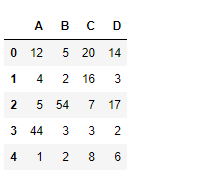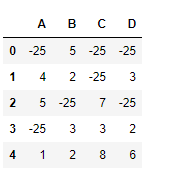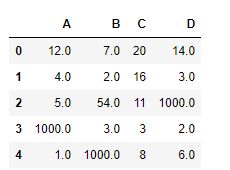Related Articles
Python | Pandas dataframe.mask()
• Last Updated : 19 Nov, 2018

Python is a great language for doing data analysis, primarily because of the fantastic ecosystem of data-centric python packages. Pandas is one of those packages and makes importing and analyzing data much easier.

Pandas` dataframe.mask()` function return an object of same shape as self and whose corresponding entries are from self where cond is False and otherwise are from other object. The other object could be a scalar, series, dataframe or could be a callable. The mask method is an application of the if-then idiom. For each element in the calling DataFrame, if cond is False the element is used; otherwise the corresponding element from the DataFrame other is used.

Syntax: DataFrame.mask(cond, other=nan, inplace=False, axis=None, level=None, errors=’raise’, try_cast=False, raise_on_error=None)

Parameters :
cond : Where cond is False, keep the original value. Where True, replace with corresponding value from other. If cond is callable, it is computed on the NDFrame and should return boolean NDFrame or array. The callable must not change input NDFrame (though pandas doesn’t check it).

other : Entries where cond is True are replaced with corresponding value from other. If other is callable, it is computed on the NDFrame and should return scalar or NDFrame. The callable must not change input NDFrame (though pandas doesn’t check it).
inplace : Whether to perform the operation in place on the data
axis : alignment axis if needed, default None
level : alignment level if needed, default None
errors : str, {‘raise’, ‘ignore’}, default ‘raise’
raise allow exceptions to be raised and ignore suppress exceptions. On error return original object. Note that currently this parameter won’t affect the results and will always coerce to a suitable dtype.

try_cast : try to cast the result back to the input type (if possible),

Returns : wh : same type as caller

Example #1: Use `mask()` function to replace all the values in the dataframe which are greater than 10 with -25

 `# importing pandas as pd``import` `pandas as pd`` ` `# Creating the dataframe ``df ``=` `pd.DataFrame({``"A"``:[``12``, ``4``, ``5``, ``44``, ``1``],``                   ``"B"``:[``5``, ``2``, ``54``, ``3``, ``2``],``                   ``"C"``:[``20``, ``16``, ``7``, ``3``, ``8``],``                   ``"D"``:[``14``, ``3``, ``17``, ``2``, ``6``]})`` ` `# Print the dataframe``df`Let’s use the `dataframe.mask()` function to replace all the values greater than 10 with -25

 `# replace values greater than 10 with -25``df.mask(df > ``10``, ``-``25``)`

Output :Example #2: Use `mask()` function with a callable. Replace all the `Na` value with 1000.

 `# importing pandas as pd``import` `pandas as pd`` ` `# Creating the dataframe ``df ``=` `pd.DataFrame({``"A"``:[``12``, ``4``, ``5``, ``None``, ``1``],``                   ``"B"``:[``7``, ``2``, ``54``, ``3``, ``None``],``                   ``"C"``:[``20``, ``16``, ``11``, ``3``, ``8``],``                   ``"D"``:[``14``, ``3``, ``None``, ``2``, ``6``]})`` ` `# replace the Na values with 1000``df.mask(df.isna(), ``1000``))`

Output :Attention geek! Strengthen your foundations with the Python Programming Foundation Course and learn the basics.

To begin with, your interview preparations Enhance your Data Structures concepts with the Python DS Course.

My Personal Notes arrow_drop_up